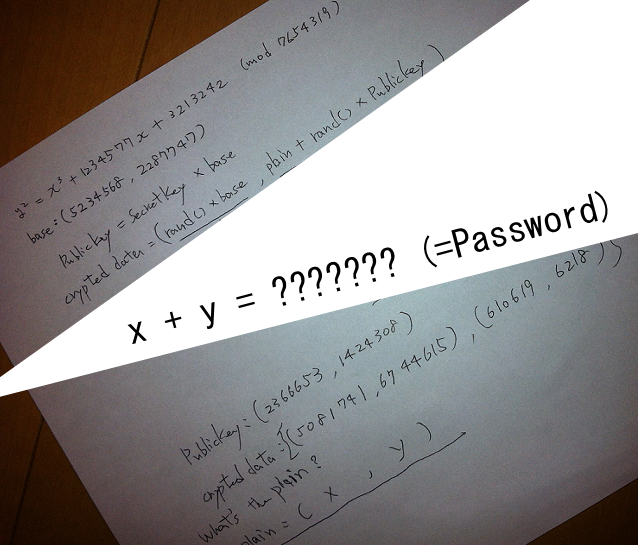# ECC¶

Warning

The current page still doesn't have a translation for this language.

Besides, you can also help to translate it: Contributing.

## 概述¶

ECC 全称为椭圆曲线加密，EllipseCurve Cryptography，是一种基于椭圆曲线数学的公钥密码。与传统的基于大质数因子分解困难性的加密方法不同，ECC依赖于解决椭圆曲线离散对数问题的困难性。它的优势主要在于相对于其它方法，它可以在使用较短密钥长度的同时保持相同的密码强度。目前椭圆曲线主要采用的有限域有

• 以素数为模的整数域GF(p)，通常在通用处理器上更为有效。
• 特征为 2 的伽罗华域GF（2^m），可以设计专门的硬件。

## 基本知识¶

$y^2+axy+by=x^3+cx^2+dx+e$

$y^2=x^3+ax+b$

$p \oplus p \oplus ... \oplus p=O$

$Q=m\cdot p =p \oplus p \oplus ... \oplus p$ （m个p参与运算）

## ECC中的ElGamal¶

### 加密¶

1. 查询用户A的公钥$E_q(a,b), q, P_a,G$
2. 在(1,q-1) 的区间内选择随机数k 。
3. 根据A的公钥计算点$(x_1,y_1)=kG$
4. 计算点$(x_2,y_2)=kP_a$ ，如果为O，则从第二步重新开始。
5. 计算$C=m+(x_2,y_2)$
6. $((x_1,y_1),C)$ 发送给A。

### 解密¶

1. 利用私钥计算点$n_a(x_1,y_1)=n_akG=kP_a=(x_2,y_2)$
2. 计算消息$m=C-(x_2,y_2)$

## 2013 SECCON CTF quals Cryptanalysis¶a = 1234577
b = 3213242
n = 7654319

E = EllipticCurve(GF(n), [0, 0, 0, a, b])

base = E([5234568, 2287747])
pub = E([2366653, 1424308])

c1 = E([5081741, 6744615])
c2 = E([610619, 6218])

X = base

for i in range(1, n):
if X == pub:
secret = i
print "[+] secret:", i
break
else:
X = X + base
print i

m = c2 - (c1 * secret)

print "[+] x:", m
print "[+] y:", m
print "[+] x+y:", m + m


[+] secret: 1584718
[+] x: 2171002
[+] y: 3549912
[+] x+y: 5720914